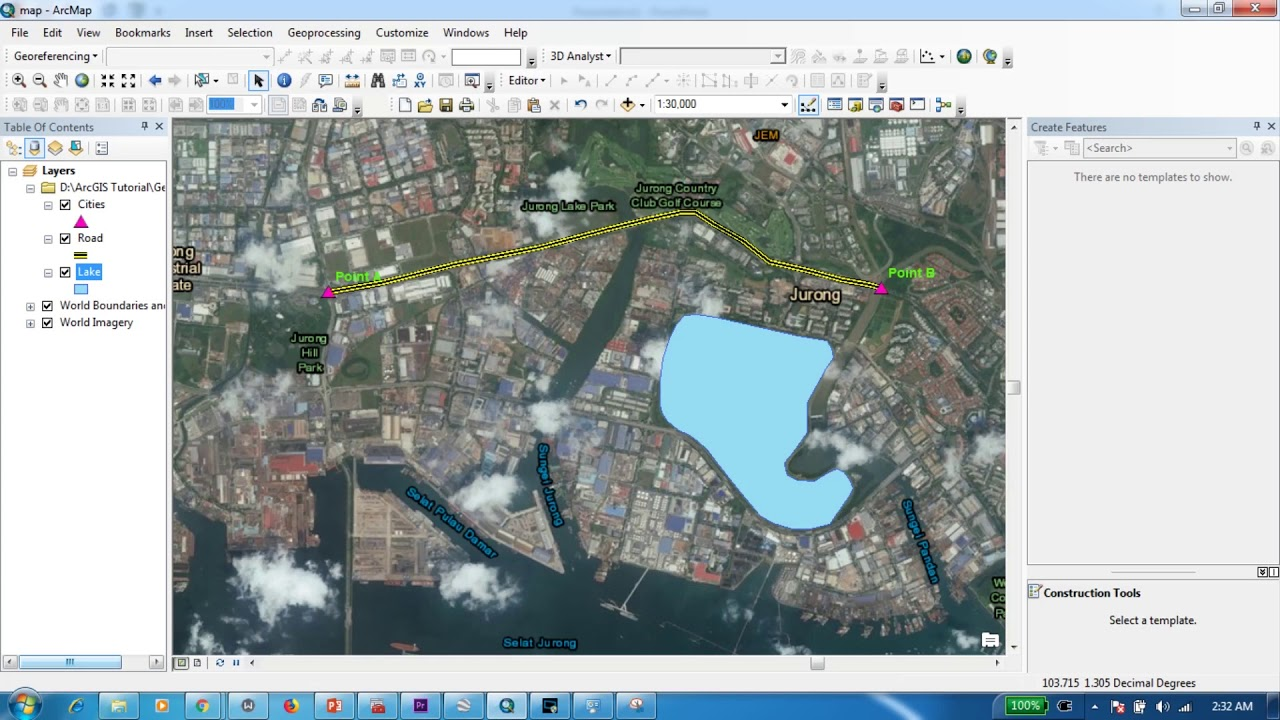# How to Calculate the Geometry of Shapefiles using ArcMap (with Real Examples)In this tutorial, I have selected an area in Singapore to calculate few geometrical properties of shapefiles. By the end of this tutorial, you will know how to calculate the area and the perimeter of a polygon, the length of a line and how to calculate the X, Y coordinates of the midpoint of a line using ArcMap tools.

In this tutorial, I have selected an area in Singapore to calculate few geometrical properties of shapefiles. By the end of this tutorial, you will know how to calculate the area and the perimeter of a polygon, the length of a line and how to calculate the X, Y coordinates of the midpoint of a line using ArcMap tools.

## Create Thiessen Polygons using ArcMap and Calculating Thiessen Weights

This tutorial emphasizes on how to create thiessen polygons using ArcMap. Furthermore, in the second half of the tutorial you will learn how to calculate the thiessen weights using a simple 'clip' operation and using the field calculator of ArcGIS

## Calculating the Volume of a Raster using 3D Analyst of ArcGIS (ArcMap + ArcScene)

Follow GeoDelta Labs on Facebook - https://www.facebook.com/geodeltalabs/This tutorial demonstrates to you how to calculate the volume of a specified river c...

## Flood Animation using 3D Analyst tools of ArcGIS (ArcScene + ArcMap)

This is a cool tutorial showing you how to do a flood inundation visualization using the 3D Analyst tools of ArcScene and ArcMap. However, it should be noted that an accurate flood inundation simulation should always be done using proper hydrodynamic tools such as HEC-RAS, MIKE 11 + MIKE 21 etc.

## How to Convert Google Earth KMZ file to Shapefile (Quick and Easy)

This tutorial is about using Google Earth to digitize a shape in the KMZ file format and exporting it into ArcMap as an ESRI Shapefile

## Importing Excel Coordinates to ArcMap and Plotting

This tutorial shows you how to import Latitude and Longitude coordinate information from excel and plot them in ArcGIS